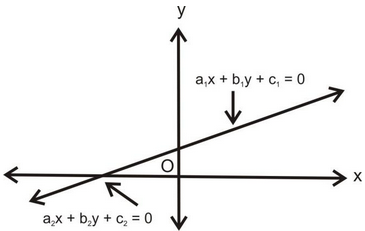# Graphical Method

## Pair Of Linear Equations In Two Variables of Class 10### GRAPHICAL SOLUTION OF LINEAR EQUATIONS IN TWO VARIABLES:

Graphs of the type (i) ax = b:

question 1. Draw the graphs of the following equations:

(i) x = 2, (ii) 2x = 1 (iii) x + 4 = 0 (iv) x = 0

Solution:

 (i) x = 2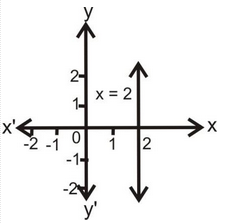(ii) 2x = 1 ⇒ x = 1/2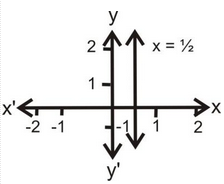(iii) x + 4 = 0 ⇒ x = –4(iv) x = 0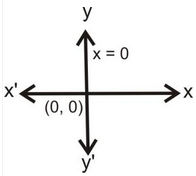Graphs of the type (ii) ay = b:

1. Draw the graphs of the following equations: (i) y = 0, (ii) y - 2 = 0, (iii) 2y + 4 = 0

 (i) y = 0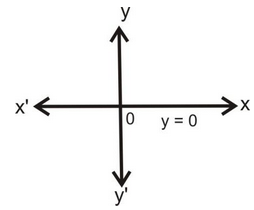(ii) y – 2 = 0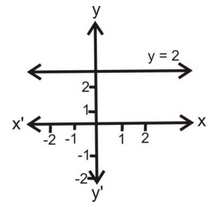(iii) 2y + 4 = 0 ⇒ y = –2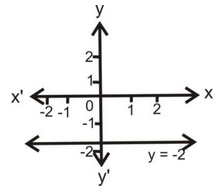Graphs of the type (iii) ax + by = 0 (Passing through origin):

question 1. Draw the graphs of the following: (i) x = y, (ii) x = –y

Solution: (i) x – y

 x 1 4 –3 0 y 1 4 –3 0

(ii) x = –y

 x 1 –2 0 y –1 2 0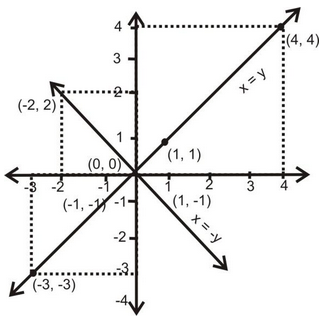Graphs of the Type (iv) ax + by + c = 0. (Making Interception x - axis, y-axis):

question 1. Solve the following system of linear equations graphically: x - y = 1, 2x + y = 8. Shade the area bounded by these two lines and y-axis. Also determine this area.

Solution: (i) x – y = 1

x – y + 1

 x 0 1 2 y –1 0 1

(ii) 2x + y = 8

(ii) 2 x + y = 8

y = 8 – 2x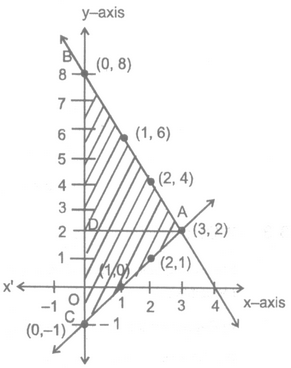X 0 1 2 Y 8 6 4

Solution is x = 3 and y = 2

Area of is x = 3 and y = 2

Area of ΔABC = 1/2 × BC × AD

= 1/2 × 9 × 3 = 13.5 Sq. unit.

### NATURE OF GRAPHICAL SOLUTION:

Let equations of two lines are a1x + b1y + c1 = 0 and a2x + b2y + c2 = 0.

(i) Lines are consistent (unique solution) i.e. they meet at one point condition is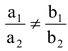.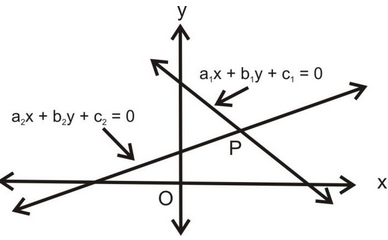(ii) Lines are inconsistent (no solution) i.e. they do not meet at one point condition is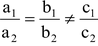.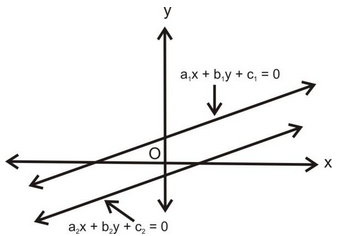(iii) Lines are coincident (infinite solution) i.e. overlapping lines (or they are on one another) condition is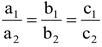.